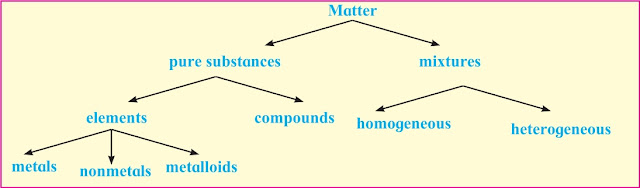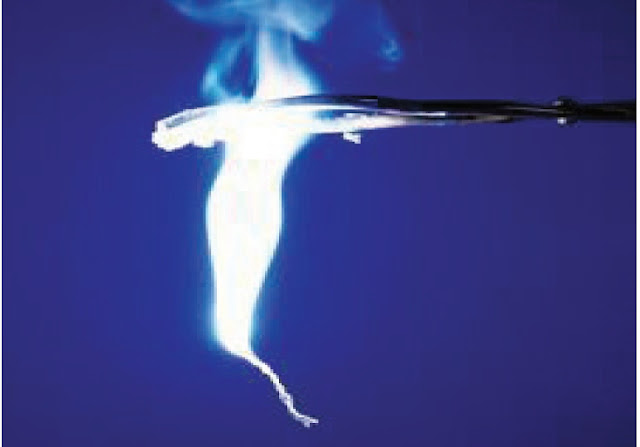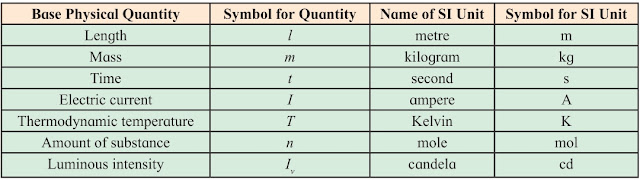# Chemistry - Some Basic Concepts of Chemistry

### Introduction :

Chemistry is the study of matter, its physical and chemical properties and the physical and chemical changes it undergoes under different conditions.

### Chemistry :

Chemistry is a central science. Its knowledge is required in the studies of physics, biological sciences, applied sciences, and earth and space sciences. The scope of chemistry is in every aspect of life, for example, the air we breathe, the food we eat, the fluids we drink, our clothing, transportation and fuel supplies,
and so on.

Though it is an ancient science, due to development and advancement in science and technology, chemistry has developed as modern science. Technological development in sophisticated instruments expanded our knowledge of chemistry which, now, has been used in applied sciences such as medicine, dentistry, engineering, agriculture and in daily home use products.

### Nature of Chemistry :

Chemistry is traditionally classified further into five branches : organic, inorganic, physical, bio and analytical. Organic chemistry is the study of the properties and reactions of compounds of carbon. Inorganic chemistry is the study of all substances which are not organic. Physical chemistry is the study of principles underlying chemistry. It deals with the studies of properties of matter. It is study of atoms, molecules, and fundamental concepts related to electrons, energies and dynamics therein. It provides basic framework for all the other branches of chemistry.

### Matter :

Matter occupies space and has mass. Matter can be further classified into pure substances and mixtures on the basis of chemical composition.Matter Diagram

Let us understand first what are pure substances and mixtures.

### Pure substances versus mixtures :

Pure substances have a definite chemical composition. They always have the same properties regardless of their origin. Mixtures have no definite chemical composition and hence no definite properties. Examples of pure substances : Pure metal, distilled water, etc.

#### Examples of mixtures :

Paint (mixture of oils, pigment, additive), concrete (a mixture of sand, cement, water)

Pure substances are further divided into elements and compounds.

### Elements :

Elements are pure substances which can not be broken down into simpler substances by ordinary chemical changes.

Elements are further classified as metals, nonmetals and metalloids.

### Metals :

i. have a lustre (a shiny appearance).
ii. conduct heat and electricity.
iii. can be drawn into wire (are ductile).
iv. can be hammered into thin sheets (are
malleable).
Examples : gold, silver, copper, iron. Mercury
is a liquid metal at room temperature.

### Non-metals :

i. have no lustre. (exception : diamond, iodine)
ii. are poor conductors of heat and electricity.
(exception : graphite)
iii. can not be hammered into sheets or drawn
into wire, because they are brittle.
Examples : Iodine, nitrogen, carbon, etc.

### Metalloids :

Some elements have properties intermediate between metals and non-metals and are called metalloids or semi-metals. Examples include arsenic, silicon and germanium.

### Compounds :

Compounds are the pure substances which can be broken down into simpler substances by ordinary chemical changes.

In a compound, two or three elements are
combined in a fixed proportion.

### Mixtures :

Mixture contains two or more substances in no fixed proportions and may be separated by physical methods. Mixtures are further divided into homogeneous and heterogeneous. Solutions are homogeneous mixtures, because the molecules of constituent solute and solvent
are uniformly mixed throughout its bulk. In heterogeneous mixtures the molecules of the constituents are not uniformly mixed throughout the bulk.
For example : Suspension of an insoluble solid in a liquid.

### States of matter :

You are also aware that matter exists in three  ifferent states namely gas, liquid and solid. In solids, constituent atoms or molecules (particles) are tightly held in perfect order and therefore solids possess definite shape and volume. Liquids contain particles close to each other and they can move around within the liquid. While in gases, the particles are far apart as compared to those in liquid and solid state. Three states of matter are interconvertible by changing the conditions of temperature and pressure.

### Properties of matter and their measurement :

Different kinds of matter have characteristic properties, which can be classified into two categories as physical properties and chemical properties.Burning of Magnesium wire
Physical properties are those which can be measured or observed without changing the chemical composition of the substance. Colour, odour, melting point, boiling point, density, etc. are physical properties. Chemical properties are the properties where substances undergo a chemical change and thereby exhibit change in chemical composition.
For example, coal burns in air to produce carbon dioxide or magnesium wire burns in air in the presence of oxygen to form magnesium oxide.

### Measurement of properties :

Many properties of matter are quantitative in nature. When you measure something, you are comparing it with some standard. The standard quantity is reproducible and unchanging. Many properties of matter such as mass, length, area, pressure, volume, time, etc. are quantitative in nature. Any quantitative measurement is expressed by a number followed by units in which it is measured. For example, length of class room can be represented as 10 m. Here 10 is the number and 'm' denotes metre-the unit in which the length is measured.

The standards are chosen orbitrarily with some universally acceped criteria. ''The arbitrarily decided and universally accepted standards are called units.''

There are several system in which units are expressed such as CGS (centimetre for length, gram for mass and second for time), FPS (foot, pound, second) and MKS (metre, kilogram, second) systems, etc.

### SI units :

In 1960, the general conference of weights and measure, proposed revised metric system, called International System of units, that is, SI units.

The metric system which originated in France in late eighteenth century, was more convenient as it was based on the decimal system. Later, based on a common standard system, the International System of Units (SI units) was established.

The SI system has seven base units as listed in Table. These are fundamental scientific quantities. Other units like speed, volume, density, etc. can be derived from these quantities.

### Physical properties :Fundamental Units
i. Mass and weight : We know that matter has mass. So mass is an inherent property of matter. It is the measure of the quantity of matter a body contains. The mass of a body does not vary as its position changes. On the other hand, the weight of a body is result of the mass and gravitational attraction. The weight of a body varies because the gravitational attraction of the earth for a body varies with the distance from the centre of the earth.

Hence, the mass of a body is more fundamental property than its weight.

The basic unit of mass in the SI system is the kilogram as given in Table. However, a fractional quantity 'gram' is used for weighing small quantities of chemicals in the laboratories. Therefore, in terms of grams it is defined (1kg = 1000 g = 103 g )

ii. Length : In chemistry we come across 'length' while express in properties such as the atomic radius, bond length, wavelenght of electromagnetic radiation, and so on. These quantities are very small therefore fractional units of the SI unit of length are used for example, nanometre (nm), picometre (pm). Here 1nm = 10-9 m, 1 pm = 10-12 m.

iii. Volume : It is the amount of space occupied
by a three dimensional object. It does not depend on shape. For measurement of volume of liquids and gases, a common unit, litre (L) which is not an SI unit is used.Litre and SI Unit of Volume
Different kinds of glassware are used to measure the volume of liquids and solutions. For example, graduated cylinder, burette, pipette, etc. A volumetric flask is used to prepare a known volume of a solution. Figure shows the types of apparatus used in laboratory for measuring volume of liquids.Volumetric glass apparatus
iv. Density : Density of a substance is its mass per unit volume. It is determined in the laboratory by measuring both the mass and the volume of a sample. The density is calculated by dividing mass by volume. It is the characteristic property of a substance.

v. Temperature : Temperature is a measure of
the hotness or coldness of an object.Thermometers of different temperature scale
There ara three common scales to measure temperature, namely
1- Degree Celsius
2- Degree Fahrenheit
3- Kelvin